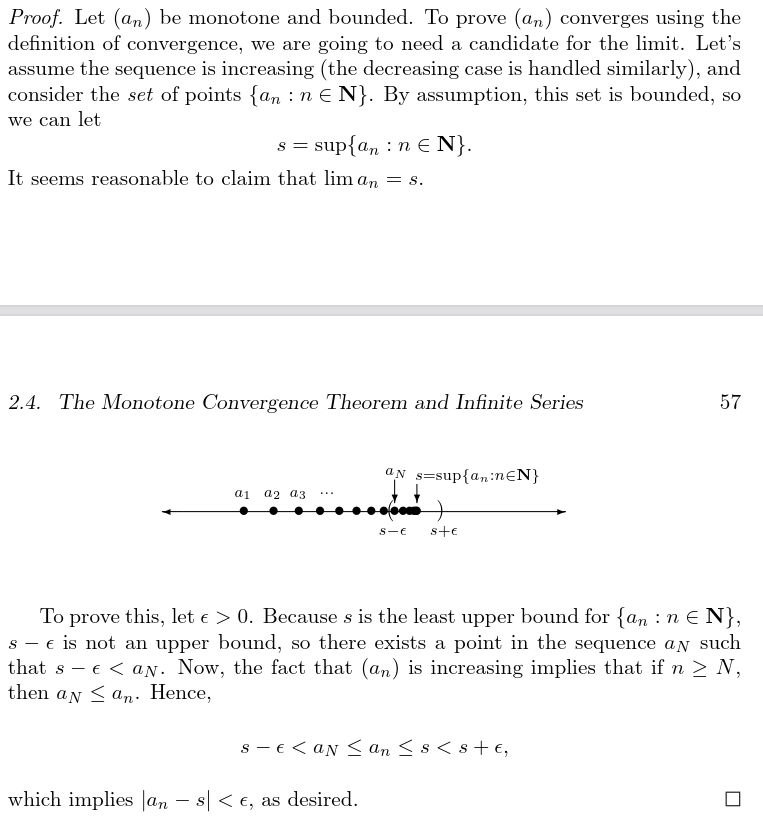Show Question
Math and science::Analysis::Tao::06. Limits of sequences

# Monotone bounded sequences converge

#### Increasing, decreasing and monotone

A sequence $$(a_n)_{n=0}^{\infty}$$ is increasing if $$a_n \le a_{n+1}$$ for all $$n \in \mathbb{N}$$ and decreasing if $$a_n \gt a_{n+1}$$ for all $$n \in \mathbb{N}$$. A sequence is monotone if it is either increasing or decreasing.

### Monotone bounded sequences converge

If a sequence is monotone and bounded, then it converges.

The easiest card ever. The proof is on the reverse; can you think of it? The proof is easy and obvious in retrospect, but it uses a property that I originally didn't consider using to solve the problem.Abbott, p57
Tao, p138